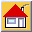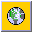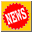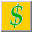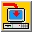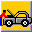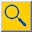Home Site Map About News Purchase Products Download Support Search
 New features in Version 4

New Functions

The new functions added in version 4.0 are listed here by category.

New Math Functions

 Name & Syntax Arguments Description & Return Value Ceil(number) number Rounds number (real) up to the nearest whole number.

Example:

Ceil (45.1)- Returns 46.0

Ceil (45.9)- Returns 46.0

 Name & Syntax Arguments Description & Return Value Floor(number) number Rounds number (real) down to the nearest whole number.

Example:

Floor (45.1)- Returns 45.0

Floor (45.9)- Returns 45.0

 Name & Syntax Arguments Description & Return Value Rand() no arguments Returns a random real number between 0 and 1.

New Contour Functions

 Name & Syntax Arguments Description & Return Value EffectiveValue (parameter_name) parameter_name The name of the parameter or layer with which contours in the current layer will be intersected. Returns the sum of areas created by the intersection of a contour and the contours in the layer parameter_name belongs to, multiplied by the respective values of these contours.

Example: In the following illustration the hatched contour belongs to a layer in which one of the parameters is assigned the expression EffectiveValue(layer_name.parameter_name), where layer_name is the layer in which the other contours reside. The hatched contour is intersected with the contours in the referenced layer and returns the value: A*10+C*10+B*20+D*30, where A, B, C, and D are the areas of intersection between the hatched contour and the contours in the referenced layer, and 10, 20 and 30 are the values of the contours in the referenced layer.

If in the referenced layer, Allow Intersection is turned on, then the function will return: A*10+C*10+B*20+D*30+B*10+D*10, since the hatched contour is also fully encompassed by the most outer contour. This would also be the case if there were additional contours fully encompassing the hatched contour.

See also EffectiveValue() for mesh nodes and elements, and grid blocks.

 The hatched contour is tested vis-a-vis all contours in the referenced layer. Polygons A, B, C and D are created by the intersection of the hatched contour and the contours in the referenced layer. The area of each intersected polygon is multiplied by the value of the contour which intersected the hatched polygon, The sum of these products is returned.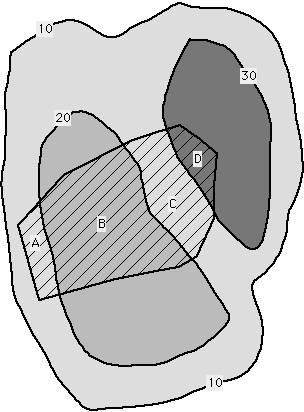New Grid Layer Functions

 Name & Syntax Arguments Description & Return Value GridAngle() Returns the angle of rotation of the grid.

New Block Functions

 Name & Syntax Arguments Description & Return Value BlockGradX (parameter_name) parameter_name Returns the X gradient calculated as central difference (real) between the value of parameter_name at the blocks to the left and right of the current block.

 Name & Syntax Arguments Description & Return Value BlockGradY (parameter_name) parameter_name Returns the Y gradient calculated as central difference (real) between the value of parameter_name at the blocks to above and below the current block.

NthColumnPos (col_num), NthRowPos(row_num)

The two functions, described in Chapter 3, have been enhanced to return the appropriate values for rotated grids. If the grid is rotated these are measured as the distance from the document's origin along the grid rotated axes.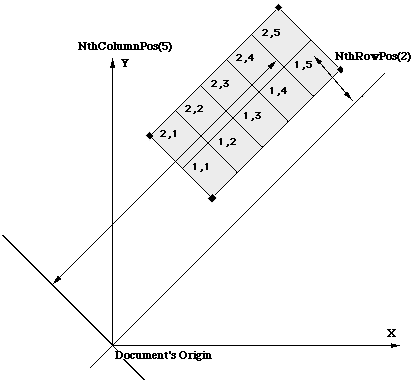New Node Functions

 Name & Syntax Arguments Description & Return Value EffectiveValue (parameter_name) parameter_name Intersects the nodes effective area with all contours in parameter_name to create a set of polygons, and returns the sum of polygons areas multiplied by their respective values.

Example: In the following illustration the hatched polygon represents the node effective area. The hatched polygon is intersected with the contours in the referenced information layer and returns the value: A*20+B*10+C*30, where A, B and C are the areas of intersection of the node effective area (hatched) and the contours in the information layer, and 10, 20 and 30 are the values of the contours in the referenced information layer.

If in the referenced layer, Allow Intersection is turned on, then the function will return: A*20+B*10+C*30+A*10+C*10, this is because the hatched polygon is also fully encompassed by the most outer contour. This would also be the case if there were additional contours fully encompassing the node's effective area.

#### Node EffectiveValue in Triangular Elements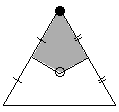Node effective area of a node in a single element.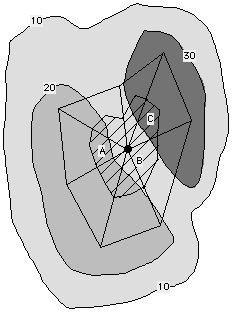The node's effective area is intersected with all contours in the referenced layer and returns the sum of polygon areas multiplied by their respective values.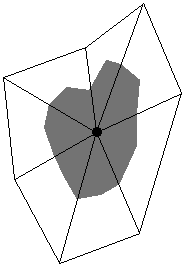Node effective area of a node common to several elements.

Node EffectiveValue in Quadrilateral Elements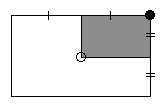Node effective area of a node in a single element.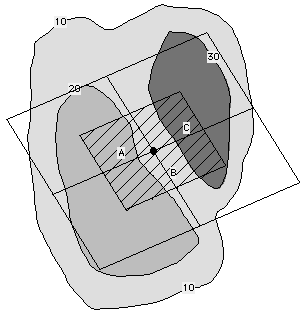The node's effective area is intersected with all contours in the referenced layer and returns the sum of polygon areas multiplied by their respective values. Node effective area of a node common to several elements.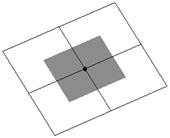Special cases: If part of the node's effective area lies outside all contours in the referenced layer, the function's return value varies with the referenced layer interpretation type. In the example below the area marked B is multiplied by:
• Nearest Contour method - The average of all adjacent contours. In this example B*(10+20)/2
• Exact Contour method - The default value of the referenced layer.
• Interpolation method - The interpolated value at the node's location.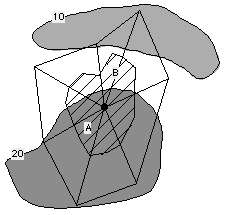The area marked B, which is not intersected by a contour, is multiplied by a different value according to the referenced layer interpretation method.

See also EffectiveValue() for contours, mesh elements and grid blocks.

To find out more about Argus and its products email marketing@argusone.com or call:
+972-9-957-5752
Tech. support: support@argusone.com
snail mail: Argus Holdings, POB 6254, Herzelia, 46160, Israel